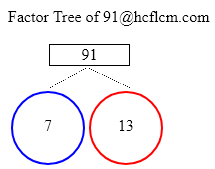# Factor Tree of 91

Make your calculations faster of finding a Factor Tree of 91 and expressing it as multiples of 7, 13 by using the Factor Tree Calculator

A factor tree is a special diagram where we find the factors of a number and then factors of those numbers until we can’t factor them anymore. In the end, what we get are all the prime factors of the original number.

Factor Tree of 91 is the list of prime numbers when multiplied results in original number 91.

Factor Tree Calculator is an online tool that displays the factors of a given number. This online handy calculator makes the calculations easy and faster for you. Enter the number in the input field. Click on the 'calculate' button and you will get the factors. It Displays the factors in a fraction of seconds.

Factor Tree of:

### Factor Tree of 91 to Calculate the Factors

 91 7 1391 = 7 x 13

Altogether expressing the number in terms of prime factors would be 7 x 13.

Learn more about Factors of 91 from here & easily calculate the factors using Factoring Calculator.

### How to find Factor Tree of 91

Splitting Numbers

We can split the number into any of its two factors. In other words, we are finding the numbers that when multiplied together give 91. Let’s start with 7 x 13 as it results in 91 on multiplying. Just like in any family tree we are now going to find the factors or ancestors of splited factors.

Let’s begin with smallest prime factor and on breaking down factor we get factors by breaking down. This could go on as divided factors are the only factors of that base factor. As per the factor tree, we would stop at the prime numbers i.e. prime factors to stop the infinite chain of the same factors.

Prime Factors

Let’s look out 91 now and we can write it as 7 x 13 and place those factors on the tree. similar to prime numbers in the initial step 7, 13 obtained here are also prime numbers and we will end up these branches.

### Factor Tree Calculations

Here are examples of Factor tree calculations.

### Frequently Asked Questions on Factor Tree of 91

1. What is factor tree?

Answer: A factor tree is a tool that breaks down any number into its prime.

2. What are the prime Factorization?

Answer: Prime Factorization is the list of prime numbers that when multiplied gives a certain product.

3. How to find a Factor Tree of 91?

Answer: Step 1:

Split the number given to factors

Step 2:

Look at the numbers and check if at least one of them is not prime.

If it is not prime repeat the process until all are prime.

∴ Factor tree of 91 is 7 x 13

4. What are the Prime Factors of 91?

Answer: Prime Factors of 91 are 7 x 13.